# Bokeh教程：基本绘图

## 导入和设置

• 使用 figure 函数创建新的绘图对象
• 使用函数 output_fileoutput_notebook（可以同时使用）告诉 Bokeh 如何显示或保存图像
• 执行 showsave 用于显示或保存绘图和布局
import numpy as np # 后面会使用，这里先导入
import pandas as pd

from bokeh.io import output_notebook, show
from bokeh.plotting import figure

output_notebook()

BokehJS 2.0.1 successfully loaded.


### 实战数据

https://raw.githubusercontent.com/CSSEGISandData/COVID-19/865c933c0f33f8ccaf4fddc45a13abdbe87036ee/csse_covid_19_data/csse_covid_19_time_series/time_series_covid19_confirmed_global.csv

df_confirmed = pd.read_csv(
"data/time_series_covid19_confirmed_global.csv",
)
df_confirmed.head()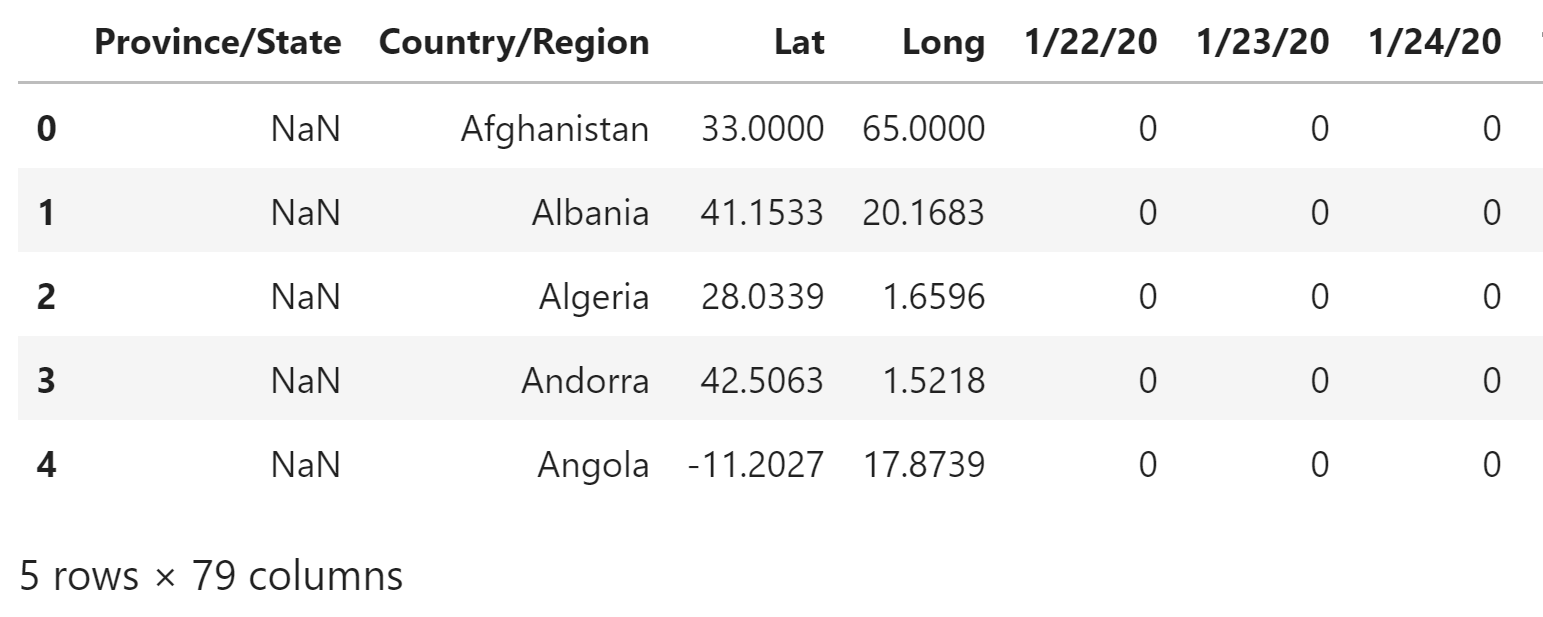原始数据集示例

df_confirmed_selected = df_confirmed.loc[
df_confirmed["Country/Region"] == "China"
].copy()
df_confirmed_selected.index = df_confirmed_selected["Province/State"]
df_confirmed_selected.drop(
["Province/State", "Country/Region", "Lat", "Long"],
axis=1,
inplace=True
)
df_confirmed_selected.columns = [
pd.to_datetime(col) for col in df_confirmed_selected.columns
]
df_confirmed_selected.head()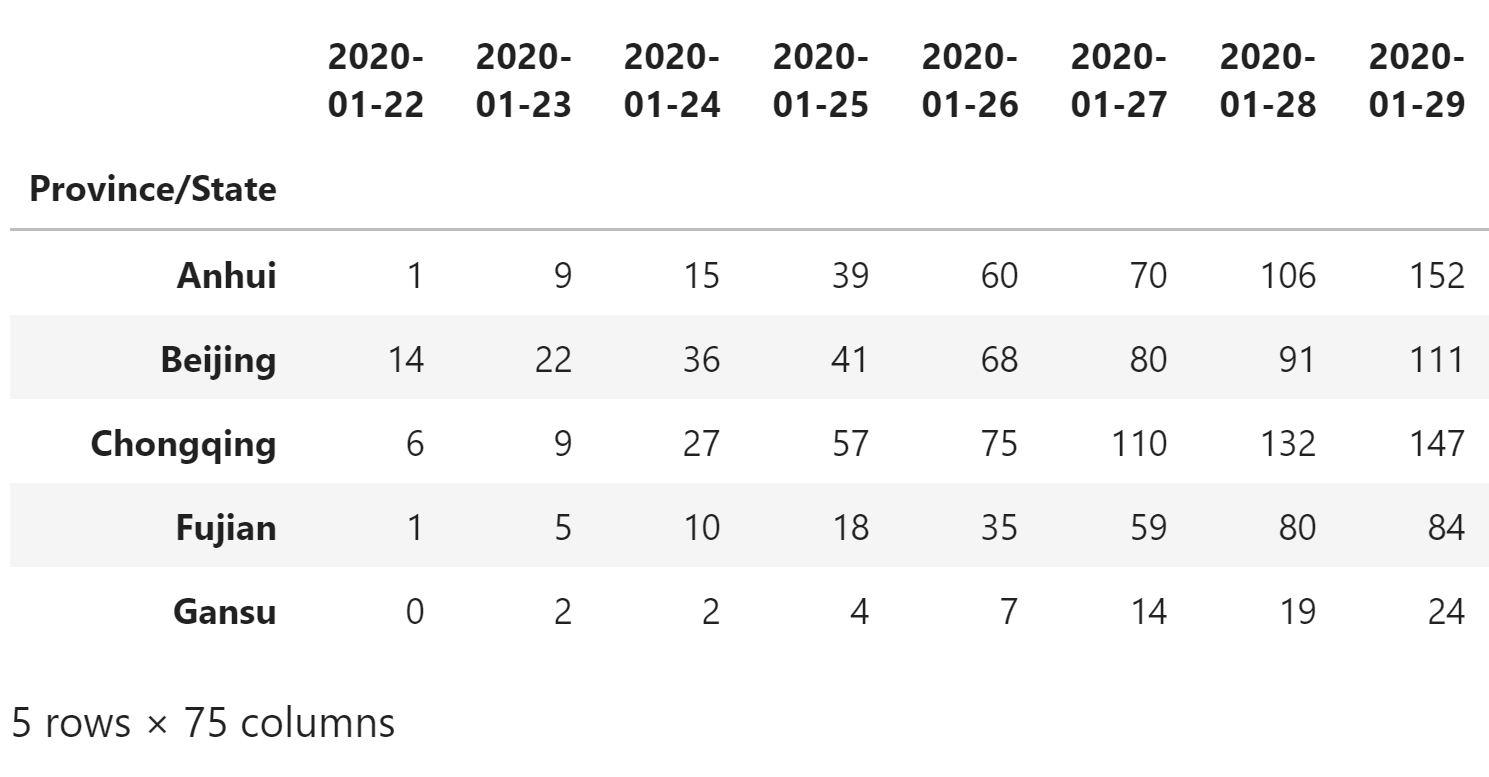调整后的数据集

df_confirmed_week = df_confirmed_selected.loc[
:, pd.to_datetime("2020-02-17"):pd.to_datetime("2020-02-23")
]
df_confirmed_week.head()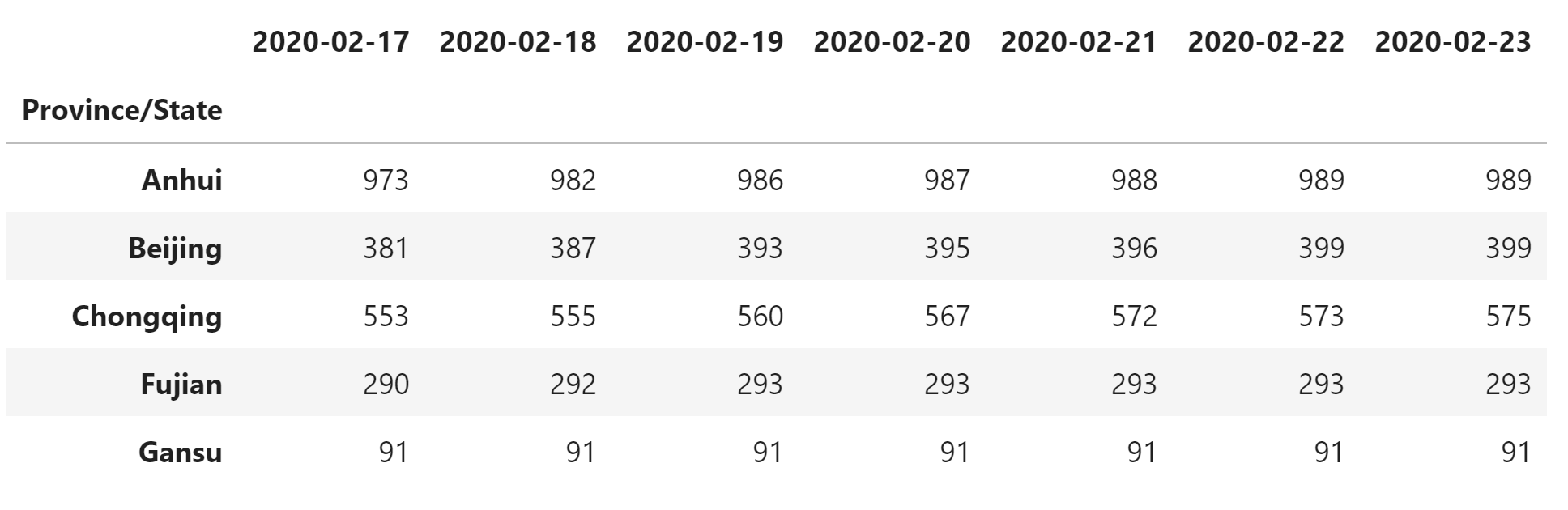一周的数据

## 散点图（Scatter Plots）

Bokeh 可以绘制许多类型的视觉形状（称为 glyphs），包括线条（lines），条形（bars），小块（pathes），六角瓦片（hex tiles）等等。 最常见的可视化任务之一是使用小 标记（marker） 符号来代表每个点，以绘制数据散点图。

• 创建一个空白的图形：p = figure(...)
• 在图形中调用一个 glyph 方法，例如 p.circle
• 调用 show 显示图形

data = df_confirmed_week[pd.to_datetime("2020-02-17")].copy()
data = data[data.between(100, 500)].sample(n=5, random_state=1)
data.index = np.arange(len(data))
data
0    146
1    121
2    457
3    238
4    130
Name: 2020-02-17 00:00:00, dtype: int64


# 使用 figure 创建使用默认工具的新图形
p = figure(plot_width=400, plot_height=400)

# 添加圆形渲染器，指定 x 和 y 坐标，大小，颜色和透明度
p.circle(
[0, 1, 2, 3, 4],  # 或使用 data_confirmed.index 代替
[146, 121, 457, 238, 130],  # 或使用 data_confirmed 代替
size=15,
line_color="blue",
fill_color="green",
fill_alpha=0.3
)

show(p) # 显示绘图结果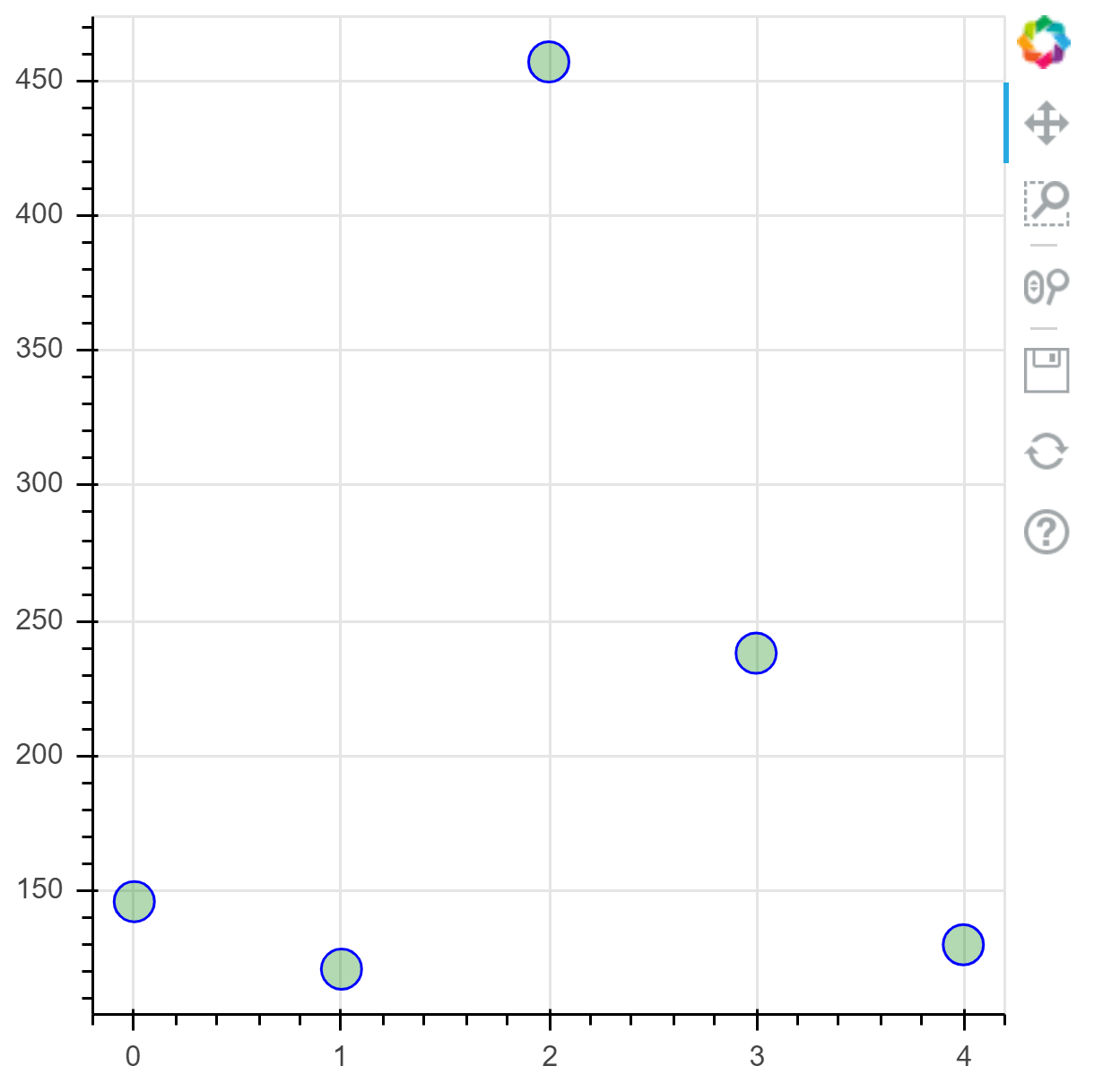圆形散点图

p = figure(plot_width=400, plot_height=400)

p.circle(
data.index,
data,
size=15,
line_color="red",
fill_color="yellow",
fill_alpha=0.8
)

show(p)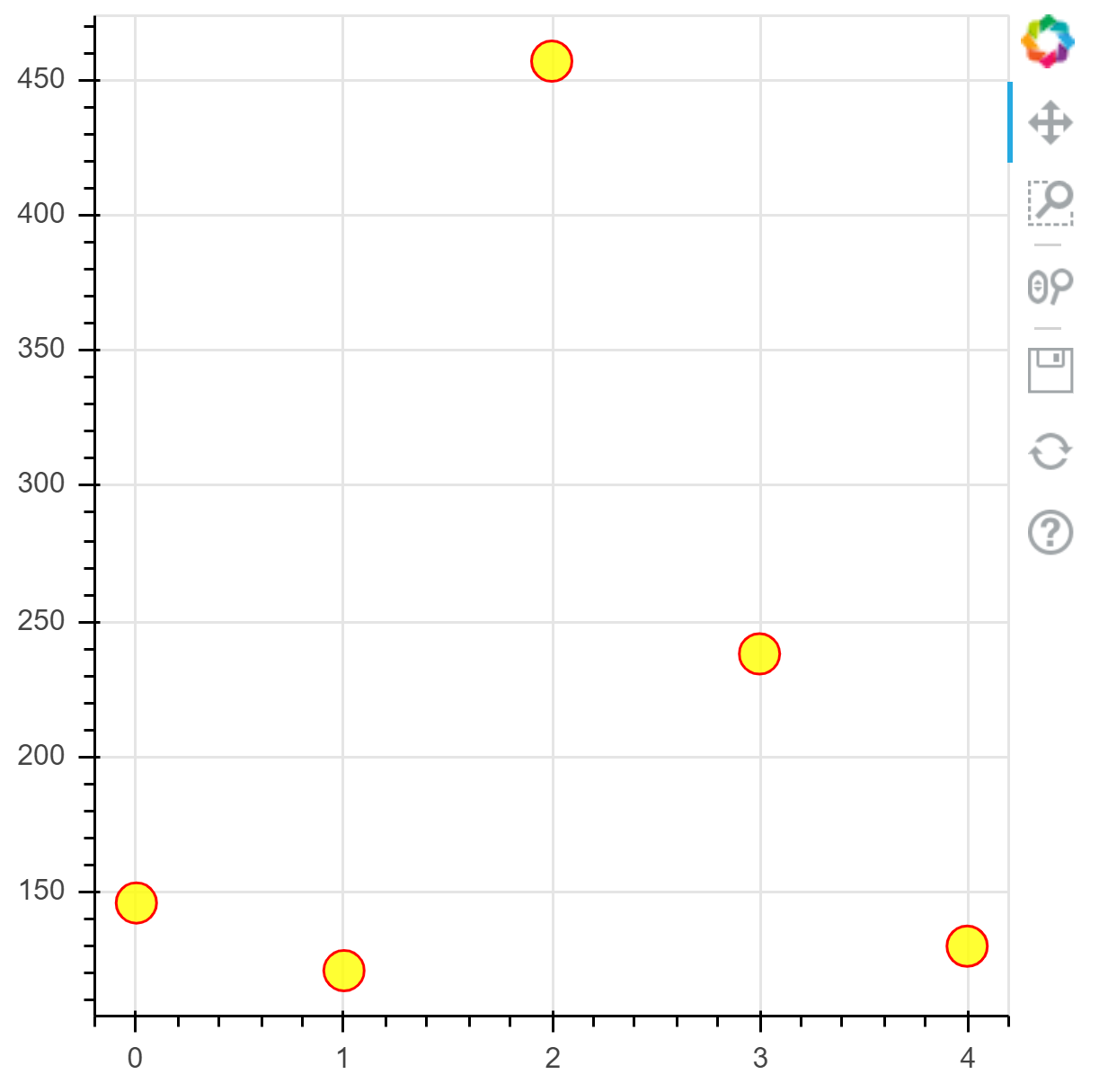修改属性后重新绘制的圆形散点图

p = figure(plot_width=400, plot_height=400)

p.square(
data.index,
data,
size=[10, 15, 20, 25, 30],
color="firebrick",
alpha=0.6,
)

show(p)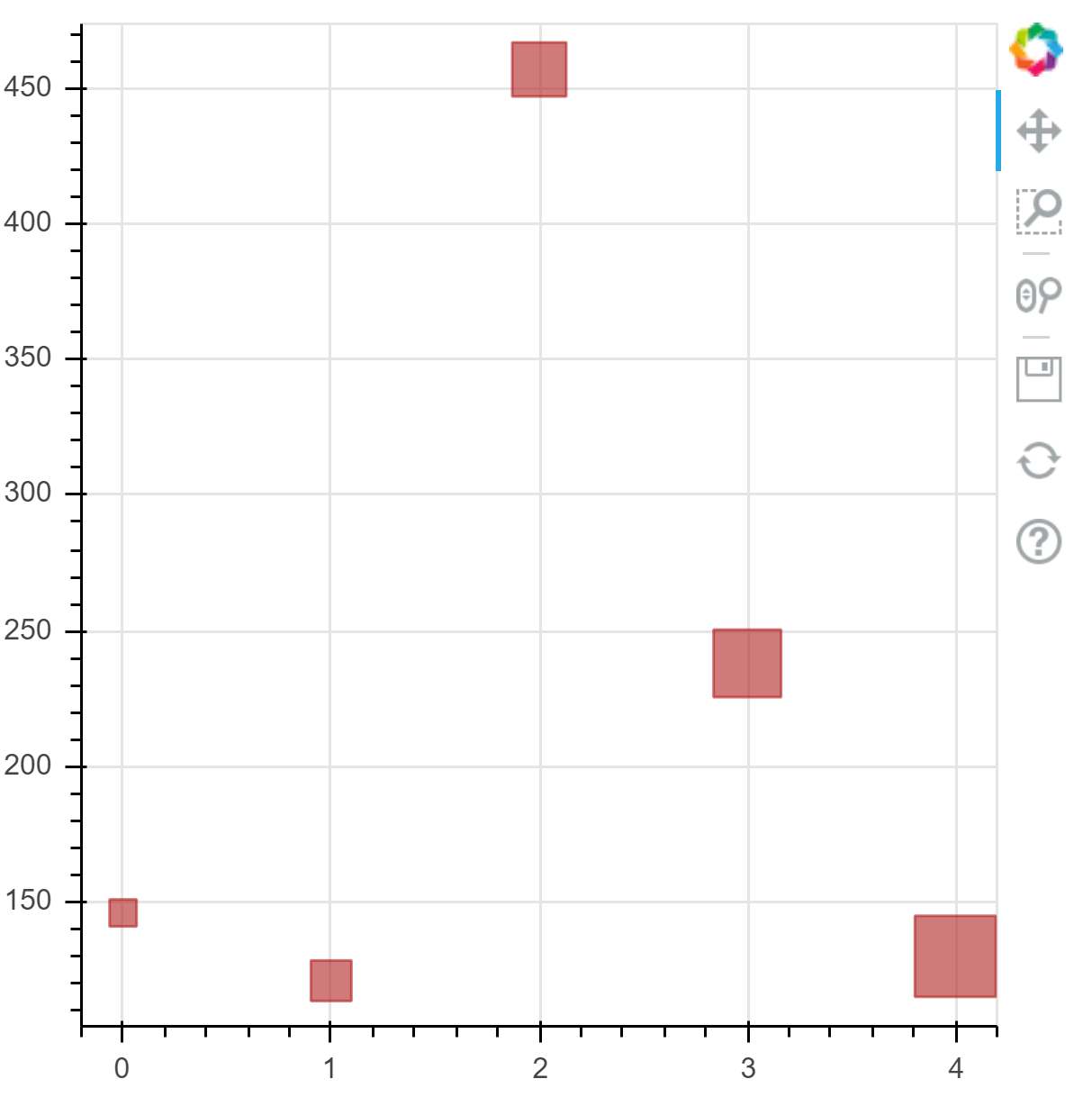正方形散点图

Bokeh 中有许多可用的标记类型，您可以在单击下面列出的参考指南条目，查看所有标记的详细信息和示例：

### 练习

https://raw.githubusercontent.com/CSSEGISandData/COVID-19/master/csse_covid_19_data/csse_covid_19_daily_reports/02-17-2020.csv

df_daily = pd.read_csv("data/02-17-2020.csv")
df_daily.head()

df_daily_a = df_daily[df_daily["Country/Region"] == "Mainland China"]
df_daily_selected = df_daily_a[df_daily_a["Confirmed"].between(100, 500)]
df_daily_selected.head()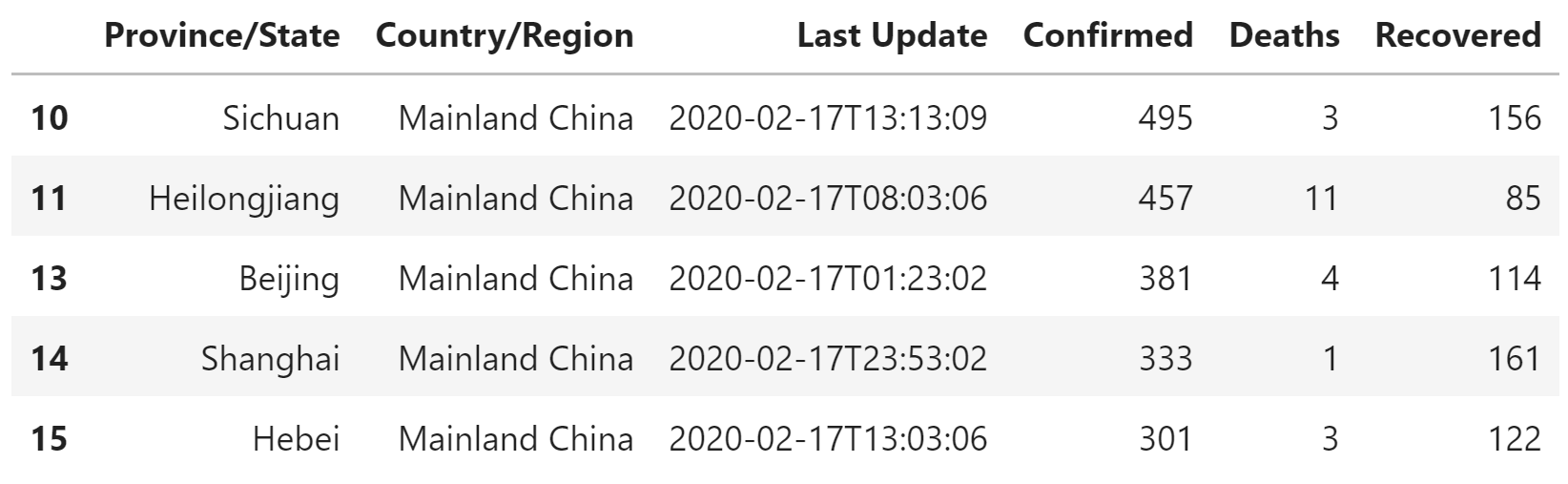筛选后的数据

p = figure(
plot_width=400,
plot_height=400,
)

p.square(
df_daily_selected["Confirmed"],
df_daily_selected["Recovered"],
color="blue",
size=8,
alpha=0.5,
)

show(p)

## 线条图

data = df_confirmed_week.loc["Beijing"]
data.index = np.arange(len(data))
data
0    381
1    387
2    393
3    395
4    396
5    399
6    399
Name: Beijing, dtype: int64

p = figure(
plot_width=400,
plot_height=400,
title="线条图"
)

# 添加线条渲染器
p.line(
[0, 1, 2, 3, 4, 5, 6],  # 或使用 data.index 代替
[381, 387, 393, 395, 396, 399, 399],  # 或使用 data 代替
line_width=2
)

show(p)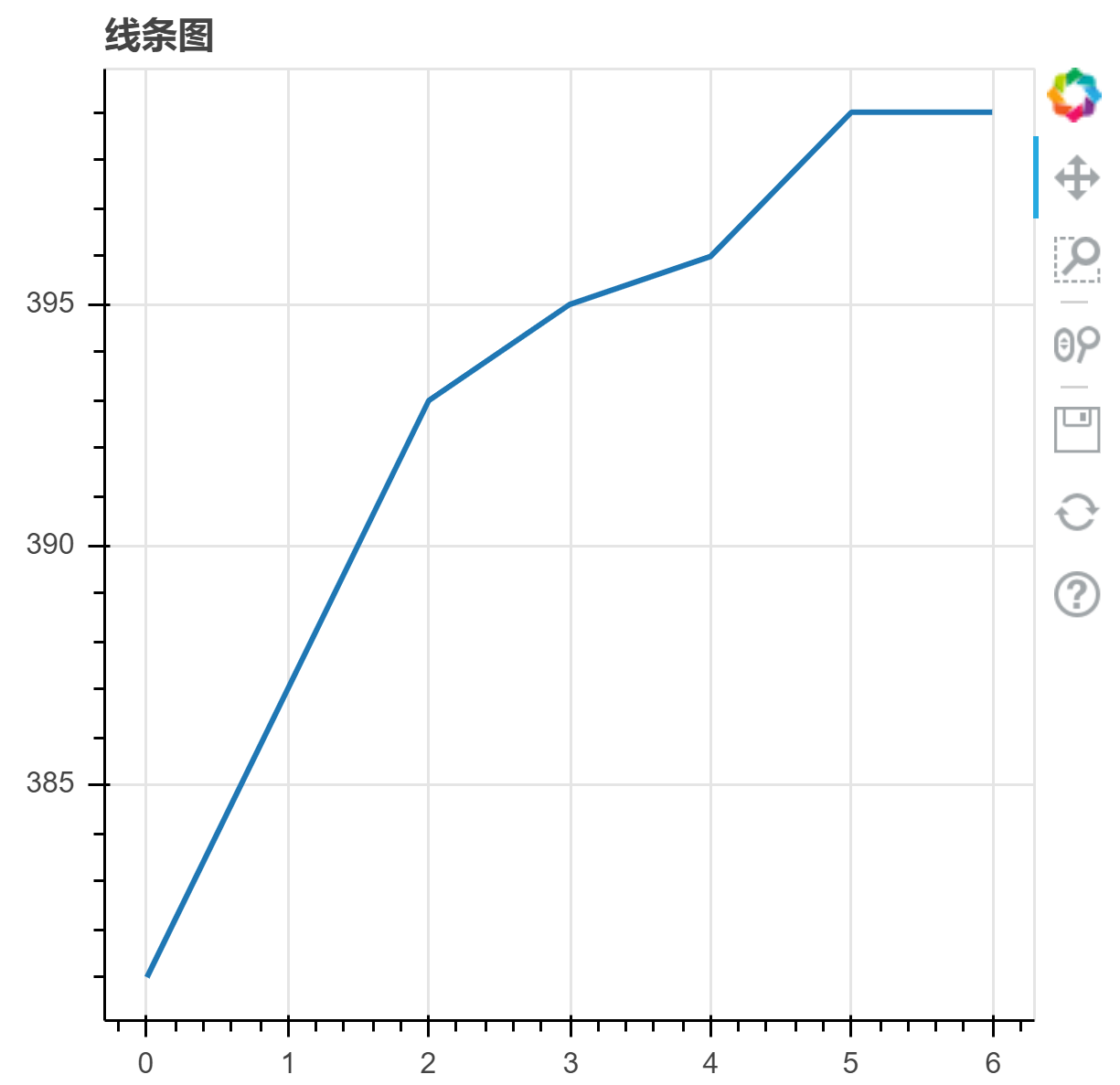线条图

p = figure(
plot_width=400,
plot_height=400,
title="使用点划线的线条图"
)

p.line(
data.index,
data,
line_width=2,
line_color="green",
line_dash="dotdash",
)

show(p)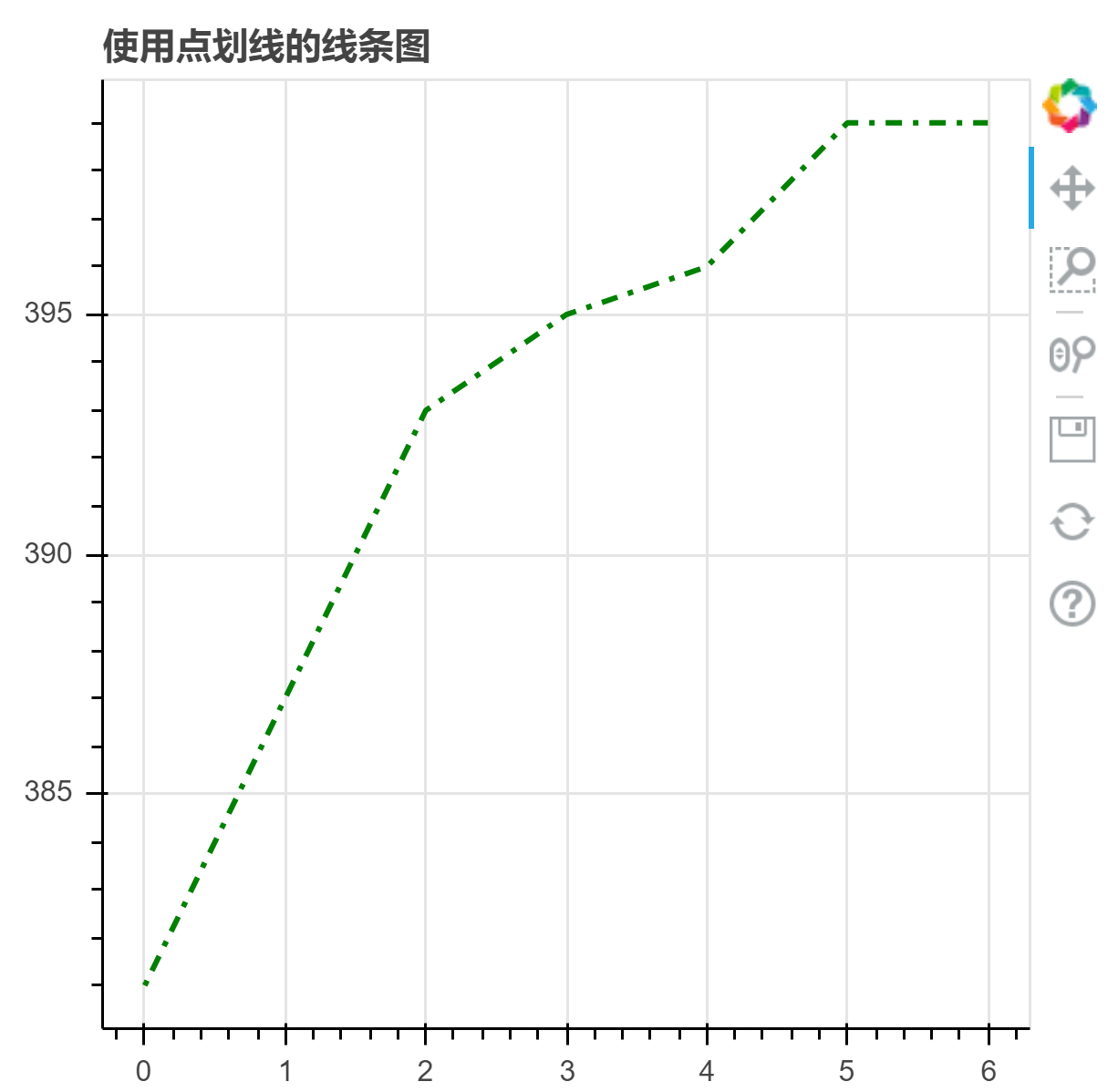使用点划线的线条图

## 日期时间坐标轴

data = df_confirmed_selected.T
data.head()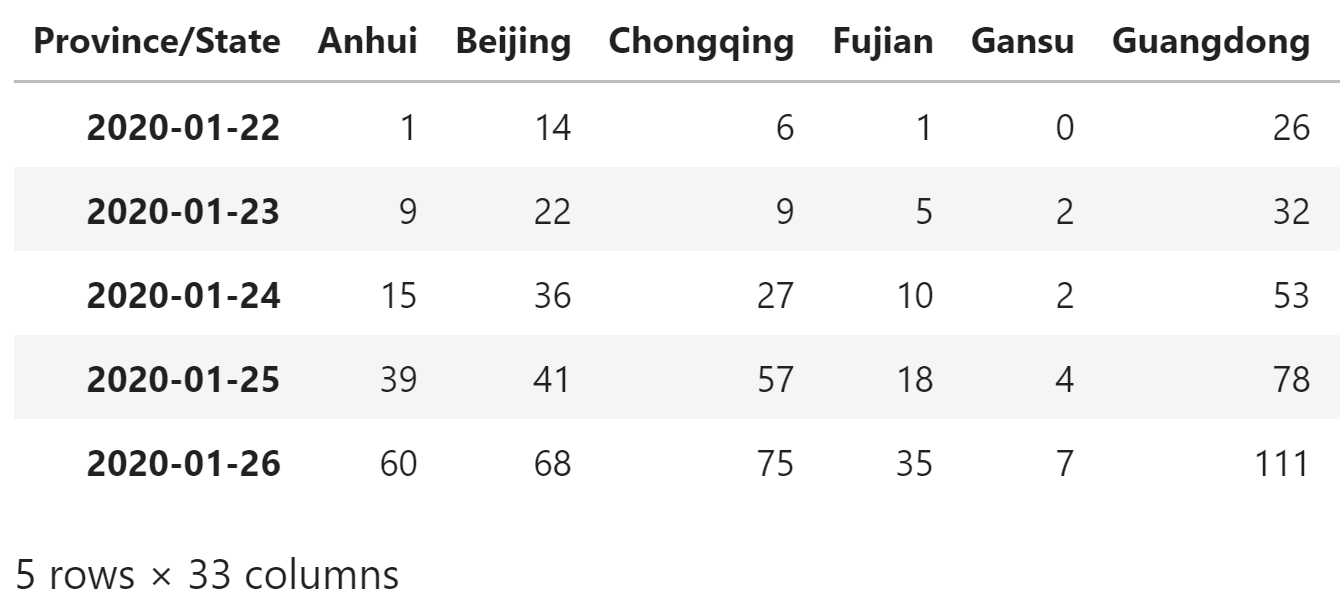转置后的示例数据表

# 选取一个月的数据
month = data.loc[pd.to_datetime('2020-02-01'):pd.to_datetime('2020-02-29')]

p = figure(
x_axis_type="datetime",
title="Confirmed",
plot_height=350,
plot_width=800
)
p.xgrid.grid_line_color=None
p.ygrid.grid_line_alpha=0.5
p.xaxis.axis_label = 'Date'
p.yaxis.axis_label = 'Case'

p.line(month.index, month.Beijing)

show(p)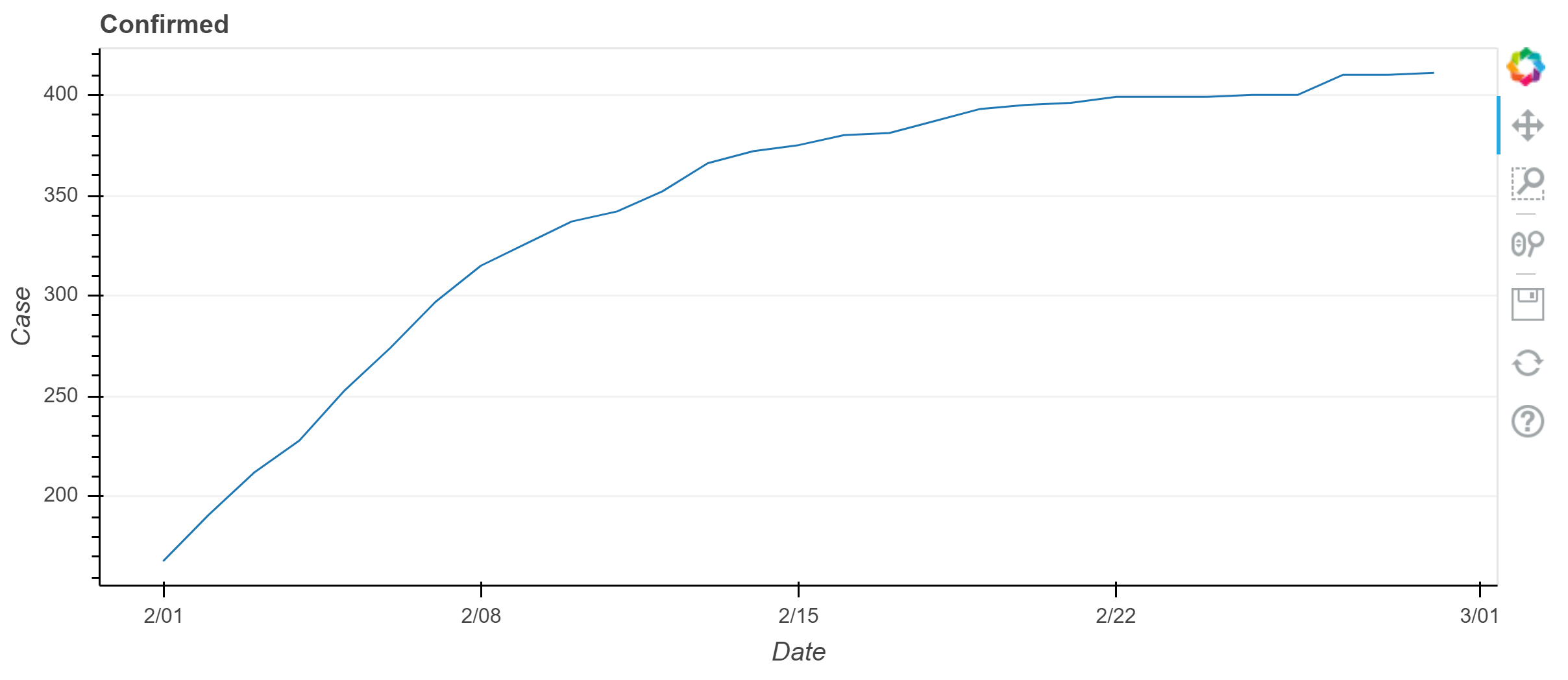日期为坐标轴的线条图

### 练习

p = figure(
x_axis_type="datetime",
title="Plot",
plot_height=350,
plot_width=800
)
p.xgrid.grid_line_color=None
p.ygrid.grid_line_alpha=0.5
p.xaxis.axis_label = 'Date'
p.yaxis.axis_label = 'Case'

p.line(
month.index,
month.Beijing,
color="green"
)
p.line(
month.index,
month.Shanghai,
color="red",
)
p.line(
month.index,
month.Tianjin,
color="blue",
)

show(p)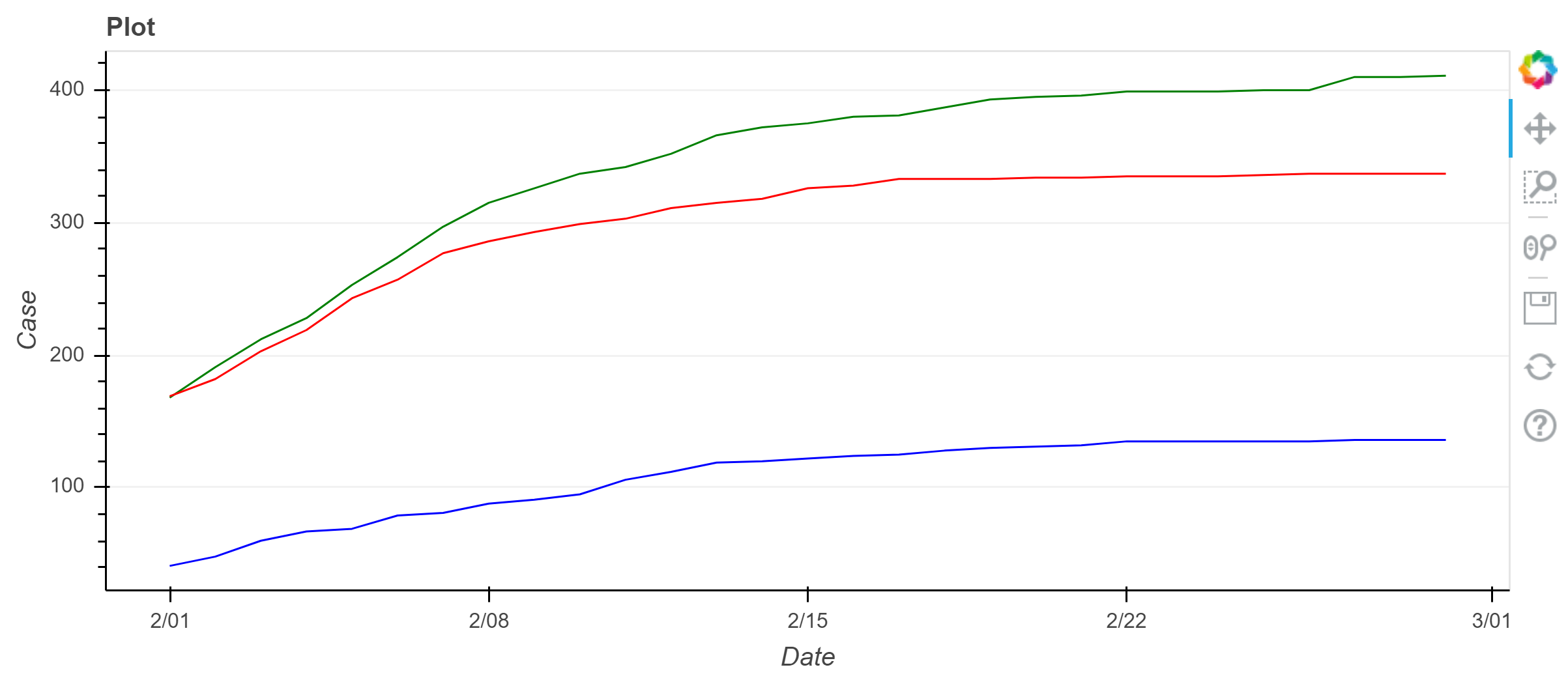多个线条图

## 六角瓦片（Hex Tiling）

Bokeh 支持使用轴向坐标hex_tile 方法绘制低级 hex tilings，如用户指南的 Hex Tiles 部分所述。 但是，六角瓦片的最常见用途之一是可视化分箱。 Bokeh 将此通用操作封装在 hexbin 函数中，该函数的输出可以直接传递到 hex_tile，如下所示。

from bokeh.palettes import Viridis256
from bokeh.util.hex import hexbin

n = 50000
x = np.random.standard_normal(n)
y = np.random.standard_normal(n)

bins = hexbin(x, y, 0.1)

# 手动为分箱分配颜色，后续将会看到如何使用 linear_cmap
color = [Viridis256[int(i)] for i in bins.counts/max(bins.counts)*255]

# match_aspect 确保无论尺寸大小，任何纬度都不会压扁
p = figure(
tools="wheel_zoom,reset",
match_aspect=True,
background_fill_color='#440154'
)
p.grid.visible = False

p.hex_tile(
bins.q,
bins.r,
size=0.1,
line_color=None,
fill_color=color
)

show(p)
# 练习: 使用 hexbin 的 size 参数进行实验，并使用不同的数据作为输入
from bokeh.palettes import Viridis256
from bokeh.util.hex import hexbin

n = 50000
x = np.random.standard_normal(n)
y = np.random.standard_normal(n)

bins = hexbin(x, y, 0.2)

color = [Viridis256[int(i)] for i in bins.counts/max(bins.counts)*255]

p = figure(
tools="wheel_zoom,reset",
match_aspect=True,
background_fill_color='#440154'
)
p.grid.visible = False

p.hex_tile(
bins.q,
bins.r,
size=0.1,
line_color=None,
fill_color=color
)

show(p)

## 图像

Bokeh 提供了两种显示图像的 glyph 方法：

• image 可以与调色板一起使用，在绘图中显示带有颜色映射的二维数据
• image_rgba 可用于在绘图中显示原始RGBA像素数据

N = 500
x = np.linspace(0, 10, N)
y = np.linspace(0, 10, N)
xx, yy = np.meshgrid(x, y)

img = np.sin(xx)*np.cos(yy)

p = figure(x_range=(0, 10), y_range=(0, 10))

# 必须为 image 参数指定矢量格式的图像数据
p.image(
image=[img],
x=0,
y=0,
dw=10,
dh=10,
palette="Spectral11"
)

show(p) 

N = 500
x = np.linspace(0, 10, N)
y = np.linspace(0, 10, N)
xx, yy = np.meshgrid(x, y)

img = np.sin(xx)*np.cos(yy)

p = figure(x_range=(0, 10), y_range=(0, 10))

p.image(
image=[img],
x=0,
y=0,
dw=10,
dh=10,
palette="Plasma256"
)

show(p)

from __future__ import division
import numpy as np

N = 20
img = np.empty((N,N), dtype=np.uint32)

# 使用数组视图分别设置每个RGBA通道
view = img.view(dtype=np.uint8).reshape((N, N, 4))
for i in range(N):
for j in range(N):
view[i, j, 0] = int(i/N*255) # red
view[i, j, 1] = 158          # green
view[i, j, 2] = int(j/N*255) # blue
view[i, j, 3] = 255          # alpha

# 使用 figure 创建一个新的绘图，使用固定的范围
p = figure(x_range=[0,10], y_range=[0,10])

# 添加一个 RGBA 图像渲染器
p.image_rgba(image=[img], x=, y=, dw=, dh=)

show(p)

from __future__ import division
import numpy as np

N = 20
img = np.empty((N,N), dtype=np.uint32)

view = img.view(dtype=np.uint8).reshape((N, N, 4))
for i in range(N):
for j in range(N):
view[i, j, 0] = int(i/N*255) # red
view[i, j, 1] = int(j/N*255) # green
view[i, j, 2] = 158          # blue
view[i, j, 3] = 255          # alpha

p = figure(x_range=[0,10], y_range=[0,10])

p.image_rgba(image=[img], x=, y=, dw=, dh=)

show(p) 

## 其它种类的 Glyphs

Bokeh 支持许多其他种类的glyphs。 您可以单击下面的用户指南链接，查看如何使用 bokeh.plotting 接口创建使用这些 glyphs 的图。

# 练习：跟随用户指南中的示例，使用其他种类 glyph 绘图。

from math import pi

p = figure(
plot_width=400,
plot_height=400
)

p.oval(
x=[1, 2, 3],
y=[1, 2, 3],
width=0.2,
height=40,
color="#CAB2D6",
angle=pi/3,
height_units="screen"
)

show(p)

## 使用多种 glyphs 绘图

# 创建一些数据
x = [1, 2, 3, 4, 5]
y = [4, 8, 2, 6, 3]

# 使用 figure 创建一个新的图形
p = figure(
plot_width=400,
plot_height=400,
)

# 添加折线图和圆点图
p.line(x, y, line_width=2)
p.circle(x, y, fill_color="white", size=8)

show(p)
# 练习: 使用多种 glyphs创建自己的图形

x = [1, 2, 3, 4, 5]
y = [6, 7, 8, 7, 3]

p = figure(
plot_width=400,
plot_height=400
)

p.vbar(
x=x,
width=0.5,
bottom=0,
top=y,
)
p.circle(x, y, fill_color="white", size=8)

show(p)

## 参考

https://bokeh.org

https://github.com/bokeh/bokeh-notebooks